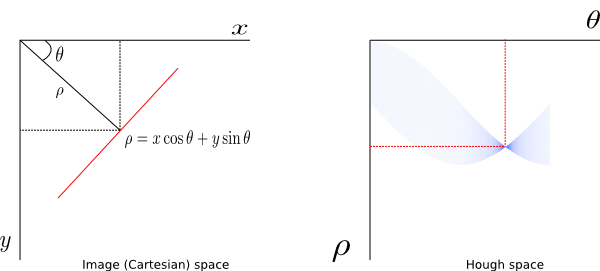# NS

## Kinematics of a Robot Car (Bicycle Model)

While going through the Sebastian Thrun‘s AI for Robotics class, I came across a programming assignment that required implementing basic kinematics of a simple robot in 2D space. The robot uses bicycle model. Here is the underlying geometry that give rise to the basic equations used in the class.Figure 1: A robot (car) movement in 2D space.

As shown in Fig. 1, the robot lies in a global cartesian coordinate space and is characterized by its  position and heading,$(x, y, \theta)$, where$\theta$ is the heading relative to$x$-axis.  The length of the robot is$L$ and its velocity along heading direction$\theta$ is$v$.

Let’s assume the robot’s origin (center of rear axle in Fig. 1) moves a distance$d$ along the heading direction. We are interested in the turn angle that the robot incurred during the movement.

## Install PyGame in OSX 10.8.5

I found very helpful instructions in this page while googling for the installing PyGame in Mac OSX 10.8.5. Saved good amount of time for me (I installed for Python 3.x):

brew install sdl sdl_image sdl_mixer sdl_ttf portmidi

brew tap homebrew/headonly

brew install smpeg --HEAD

pip3 install hg+http://bitbucket.org/pygame/pygame

## Linear Hough Transform Using Python

In this post I will explain the Hough transform for line detection. I will demonstrate the ideas in Python/SciPy.

Hough transform is widely used as a feature extraction tool in many image processing problems. The transform can be used to extract more complex geometric shapes like circles and ellipses but this post focuses on extracting lines in an image.

Quick Conceptual Review

In Cartesian coordinates, a line can be represented in slope-intercept form as$y=mx+c.$

The left panel in Fig. 1 shows a line (red color) in Cartesian coordinate system. The y-axis is chosen to be positive downward so that it matches with the commonly used image coordinate convention (top-left corner of an image is its origin). The value of$\theta$ is assumed positive when measured in clockwise direction from x-axis (to make the results comparable to MATLAB).Fig. 1: Representation of a line in Cartesian (left) and Hough (right) coordinates.

The equation of the line can be modified to get its alternate representation as$\rho=x\cos\theta+y\sin\theta$,

where$\rho$ is the distance of the line from the origin and and$\theta$ is the angle of the vector, of least magnitude,  to the line. The transformed coordinate space which has$\theta$ and$\rho$ as its axes, as shown in right panel of the above figure, is called Hough space. A point in image space is mapped to a sinusoidal curve in Hough space. A set of points belonging to a line in image space get mapped to a set of sinusoids intersecting at a point in Hough space. So the problem of detecting a line in an image becomes a problem of detecting a point in Hough space. Continue reading

## Restore GNOME Panel in Ubuntu

If you accidentally removed the panel in Ubuntu (I believe it should work with any distro with GNOME desktop), follow these steps to restore the default GNOME panel:

Open terminal (if there is no place to click, press Alt-F2 and run gnome-terminal).

Run:

gconftool-2 –shutdown (OR gconftool –recursive-unset /apps/panel)

rm -r  ~/.gconf/apps/panel

pkill gnome-panel

You should get your default panel back.

(Ref: www dot watchingthenet dot com)